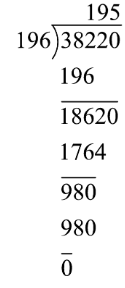# Find the HCF of number 196 and 38220.Verified
125.6k+ views
Hint- To solve this problem we will use Euclid’s algorithm which states that the greatest common divisor of two numbers is obtained by dividing the greater number by smaller number and if remainder remains then divide smaller number by the remainder and repeat this process till number is not exactly divisible.

Complete step-by-step solution -
Given numbers are 196 and 38220
Hence, 38220>196
Using Euclid’s division algorithm, we will divide 38220 by 196Hence, the number 38220 is exactly divisible by 196 so HCF is 196.

Note- The Euclid’s division algorithm is the fastest method to determine HCF of any number. The HCF can also be found using factors of numbers. First step is factorising the given number. Second take the common terms from both the factors of numbers. At last multiply all the common terms and hence the result is the HCF of that number.